## Killing with flowers [Killer Sudoku]

For fans of Killer Sudoku, Samurai Sudoku and other variants

### Re: Killing with flowers [Killer Sudoku]

Mathimagics wrote:A challenge therefore suggests itself - making puzzles that are even worse! How far can we go, making valid puzzles that take even more time for software solvers?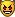That is awful because of how time consuming it would be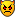I will try to dig up the worst case I posted to see how it compares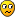tarek

Posts: 3759
Joined: 05 January 2006

### Re: Killing with flowers [Killer Sudoku]

This was posted almost 11 years ago so don't hate me if turns out to be easy according to your standards.

It was from an "unsolvable list" designed as machine defeating - at the time -
http://rcbroughton.co.uk/sudoku/forum/viewtopic.php?f=3&t=434
this is the one that took the longest

Unsolvable 41code: Show
3x3::k:7168:7168:4866:4866:4866:6149:6149:6149:5128:4873:7168:7168:7168:4866:4866:6149:5128:5128:4873:4873:5908:5908:5908:5908:4888:5128:6682:4873:7196:5908:6174:4888:4888:4888:5128:6682:7196:7196:8230:6174:6174:6174:4888:6682:6682:7196:6190:8230:8230:8230:6174:7475:6682:4917:7196:6190:8230:7475:7475:7475:7475:4917:4917:6190:6190:5697:5442:5442:7236:7236:7236:4917:6190:5697:5697:5697:5442:5442:5442:7236:7236:

tarektarek

Posts: 3759
Joined: 05 January 2006

### Re: Killing with flowers [Killer Sudoku]

Tarek's example is definitely harder for both SumoCue and JSudoku:

Code: Select all
` Case                        JSudoku   SumoCue --------------------------------------------- Wecoc Sample puzzle            330s      280s Tarek "Unsolvable #41"         611s      825s`

SumoCue is a pain to test because it doesn't report any information on the solving process. It needs an observer with a stopwatch to get the time.

I don't expect to be able to find any harder examples until I have a suitably efficient and capable solver, which can then explore sum/cage perturbations, looking for harder puzzles.Mathimagics
2017 Supporter

Posts: 1870
Joined: 27 May 2015
Location: Canberra

### Re: Killing with flowers [Killer Sudoku]

From memory: I tried to explore those difficult puzzles by constructing the the busiest starting position (Number of candidates out of 729). This was achieved using cages with number of cells having the most possibilities then going further with perturbations that result in cages with a cell number / sum with the most possibilities. Unsolvable #41 is an example towards that. If you find a way to rate them faster, you will find more difficult puzzles no doubt.

tarektarek

Posts: 3759
Joined: 05 January 2006

### Re: Killing with flowers [Killer Sudoku]

Weccoc, you a made mistake with your last 4 hidden cages, sum values 36, 42, 42, 48 don't match with the solution from Mathimatics.
creint

Posts: 335
Joined: 20 January 2018

### Re: Killing with flowers [Killer Sudoku]

creint wrote:Weccoc, you a made mistake with your last 4 hidden cages, sum values 36, 42, 42, 48 don't match with the solution from Mathimatics.

Fixed. Sum values were ok but I forgot to paint one cell in each, sorry. Well spotted!

tarek wrote:I had a play with creating “the most difficult killer” before.

Very nice one tarekCurious how SumoCue wins with mine, but JSudoku wins with yours.

Mathimagics wrote:Curiously, JSudoku reports "Unique solution found with 35 guesses" ??Maybe that would be a better way to rate them?Or the number the of nodes traversed...
Processing time is computer-dependent, that's why I would avoid using that.

I have a few other "very hard" ones like that one, I'll be testing them later.
Wecoc

Posts: 76
Joined: 08 April 2019
Location: Girona, Catalonia

### Re: Killing with flowers [Killer Sudoku]

Wecoc wrote:Maybe [guesses] would be a better way to rate them?Or the number the of nodes traversed...
Processing time is computer-dependent, that's why I would avoid using that.

Adding a few more samples from Tarek's collection demonstrates that "guesses" reported by JSudoku is poorly correlated with time, and that the "number of nodes" is much better:

Code: Select all
` Case                       JS: Guess        Nodes   Time -------------------------------------------------------- Tarek "Unsolvable  #1"           28        227086     6s Tarek "Unsolvable #40"           27       4416433     9s Tarek "Unsolvable #42"           38     110450415   240s Wecoc Sample puzzle #1           35     182765067   409s Tarek "Unsolvable #41"           36     337230898   762s`

Relative times on same machine, using same software are still a useful indicator …. note also that the times I first reported for our two best cases - 330s and 611s - turned into 409s and 762s when I retested them to get the node counts!Mathimagics
2017 Supporter

Posts: 1870
Joined: 27 May 2015
Location: Canberra

### Re: Killing with flowers [Killer Sudoku]

Rating involves Resolution rules and how to apply them: (breadth 1st or depth 1st). There is an issue with how the solver/rater responds when encountering several isomorphic versions of the same puzzle. If the solver / rater varies wildly then it’s bad news I’m afraid.

If we get to the guessing phase (recursion in one way or another) then again the solver / rater has to stay consistent with isomorphic versions or it could fall foul of the worst case scenario.

tarek

[Added:] Just Checked on JS 3 versions of Unsolvable #40:
Code: Select all
`Unsolvable #40Original:Starting to Check Grid Validity. Be patient this may take several minutes for hard grids...Check Grid Validity done in 8592 milliseconds4415293 nodes traversedUnique solution found with 27 guesses90 degrees clockwise rotation:Starting to Check Grid Validity. Be patient this may take several minutes for hard grids...Check Grid Validity done in 7953 milliseconds3787459 nodes traversedUnique solution found with 31 guesses180 degrees rotation:Starting to Check Grid Validity. Be patient this may take several minutes for hard grids...Check Grid Validity done in 11031 milliseconds5429720 nodes traversedUnique solution found with 32 guesses`

One way to normalise this is to average the result over a certain number of random transformations (well in this case all the transformations are 8) which is similar to what Suxratt does! the problem is that this will again prolong an already time-consuming process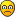tarek

Posts: 3759
Joined: 05 January 2006

### Re: Killing with flowers [Killer Sudoku]

tarek wrote:Rating involves Resolution rules and how to apply them: (breadth 1st or depth 1st). There is an issue with how the solver/rater responds when encountering several isomorphic versions of the same puzzle. If the solver / rater varies wildly then it’s bad news I’m afraid.

This is because the solver starts the guessing from the first cell and continues until it finds the next useful cell, right?

On the Ruby-based solver I made a few ago for default sudoku , I kind of solved this by defining which ones should be looked first.
It sorts the cells by the number of candidates they have, starting with the lowest. That also accelerates the process a bit.
Many cells will have the same number of possible candidates: in that case those are still ordered by coordinate so it's not totally fixing the problem, but it may considerably reduce the variability between isomorphs.

Piece of code: Show
Code: Select all
`  table = []  for iy in 0...9    for ix in 0...9      # Get each cell (ordered by coordinate)      cell = get(ix, iy)      # Don't include if it's already solved      next if cell.values.size == 1      # Store in table with this format: [[X, Y], candidates size]      table.push([[ix, iy], cell.values.size])    end  end  # Sort by candidates size (lowest first)  table.sort!{|a,b| a - b}  # Check every cell based on the new sorting  for t in 0...table.size    current_cell = get(*table[t])    # (...)  end`

Sorry if I'm talking nonsense, there might be better ways to approach this.

--

Good news! I found a harder flower, but it's still easier than tarek's Unsolvable #41.
Even if it's computally harder to process than first one, houses, pseudo-houses and similar tricks might be a bit more useful in this one.

Flower #2

Code: Select all
`.--.-----.-----------.-----.|10|20   |25         |11   ||  |     |  .--------+-----:|  |     |  |20      |20   |:--+--.--'--'--.  .--:     ||25|20|25      |  |25|     ||  |  '--.  .--'--'  :-----:|  |     |  |        |25   ||  |  .--:  :--.--.  :--.  ||  |  |25|  |2 |25|  |20|  ||  '--:  '--'--:  :--'  |  ||     |        |  |     |  |:-----:  .--.--'  '--.  |  ||21   |  |20|        |  |  ||     :--'  '--.--.--'--+--:|     |        |25|20   |11|:-----+--------'  |     |  ||10   |           |     |  |'-----'-----------'-----'--'`

Code: Select all
`3x3::k:2561:5122:5122:6403:6403:6403:6403:2820:2820:2561:5122:5122:6403:5125:5125:5125:5126:5126:6410:5129:6408:6408:6408:5125:6407:5126:5126:6410:5129:5129:6408:6407:6407:6407:6415:6415:6410:5129:6411:6408:524:6413:6407:5134:6415:6410:6410:6411:6411:6411:6413:5134:5134:6415:5397:5397:6411:5139:6413:6413:6413:5134:6415:5397:5397:5139:5139:5139:6418:5137:5137:2832:2580:2580:6418:6418:6418:6418:5137:5137:2832:`

Code: Select all
`724524 ms (463173 ms on Flower #1)254362226 nodes traversedUnique solution found with 39 guesses`
Wecoc

Posts: 76
Joined: 08 April 2019
Location: Girona, Catalonia

### Re: Killing with flowers [Killer Sudoku]

Code: Select all
` Case                       JS: Guess        Nodes   Time -------------------------------------------------------- Tarek "Unsolvable #42"           38     110450415   240s Wecoc Sample puzzle  #1          35     182765067   409s Wecoc Sample puzzle  #2          39     254326421   455s Tarek "Unsolvable #41"           36     337230898   762s`

Puzzle rating is a notoriously complex and inexact science. Puzzle ranking using a particular solver is the best we can do with these puzzles, I think.

Wecoc wrote:On the Ruby-based solver I made a few ago for default sudoku , I kind of solved this by defining which ones should be looked first.
It sorts the cells by the number of candidates they have, starting with the lowest. That also accelerates the process a bit.
Many cells will have the same number of possible candidates: in that case those are still ordered by coordinate so it's not totally fixing the problem, but it may considerably reduce the variability between isomorphs.

Most DFS (depth-first search) use some form of this. The problem of "which cell next?" (the next cell selection strategy) is itself an area of complexity, even for standard Sudoku. What to do when you have many cells with the least NPV (number of possible values)?

Your puzzles start with one given, which eliminates a single value in row and column. Some other single eliminations can be made from the 2-cell cages, but we still wind up with 29 choices for "best cell" candidate, each with NPV = 8.

With Killers, much like Kakuro, we need to look beyond single cell candidates. Tarek's #41 puzzle offers more candidate eliminations, but it has no small cages. A cage of size 2 will always be better because you know any guess will immediately force 2 values, so the next cell strategy needs to be more subtle.

The isomorphic variations Tarek mentioned above will always occur when there is more than one cell with minimum NPV. Most solvers will simply take the first cell in the list, there being no other way to distinguish between them. Rotating/reflecting the original puzzle will result in an essentially-different cell being chosen, and this might be a better choice, or a worse one.Mathimagics
2017 Supporter

Posts: 1870
Joined: 27 May 2015
Location: Canberra

### Re: Killing with flowers [Killer Sudoku]

We have a new leader!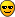I took Wecoc's first example, and tested the 4 ways that the central cell can be combined with a neighbouring cage. Only one of these produced a valid puzzle (modification A), which is a leader in its own right. Modification B, while it has multiple solutions, is even "better", taking the longest time of all to find the first 2 solutions.

Modification A: 1(1) + 5(2) => 6(3)
Wecoc 1a: Show
Code: Select all
`3x3::k:6144:6144:6401:6401:6401:6658:6658:6658:6658:6144:6401:6401:5123:4868:4868:4868:6661:6658:6144:5126:5126:5123:5123:5123:4868:6661:6661:6144:5126:5127:3336:2313:2313:5130:5130:6661:6155:5126:5127:3336:1555:1555:5130:5133:6661:6155:5127:5127:4366:4366:1555:5130:5133:6671:6155:6155:5392:5137:5137:5137:5133:5133:6671:6162:6155:5392:5392:5392:5137:6412:6412:6671:6162:6162:6162:6162:6412:6412:6412:6671:6671:`

Modification B: 1(1) + 17(2) => 18(3)
Wecoc 1b: Show
Code: Select all
`3x3::k:6144:6144:6401:6401:6401:6658:6658:6658:6658:6144:6401:6401:5123:4868:4868:4868:6661:6658:6144:5126:5126:5123:5123:5123:4868:6661:6661:6144:5126:5127:3342:2312:2312:5130:5130:6661:6155:5126:5127:3342:4627:1289:5130:5133:6661:6155:5127:5127:4627:4627:1289:5130:5133:6671:6155:6155:5392:5137:5137:5137:5133:5133:6671:6162:6155:5392:5392:5392:5137:6412:6412:6671:6162:6162:6162:6162:6412:6412:6412:6671:6671:`

Code: Select all
` Case                       JS: Guess        Nodes   Time -------------------------------------------------------- Tarek "Unsolvable #42"           38     110450415   240s Wecoc Sample puzzle  #1          35     182765067   409s Wecoc Sample puzzle  #2          39     254326421   455s Tarek "Unsolvable #41"           36     337230898   762s Wecoc #1 mod A                   37     535023523   967s Wecoc #1 mod B                    ?    1557326455  2825s  (MS)`

The guess count for mod B is missing because I was using "Check Grid Validity" (rather than "Recursively Solve") to ensure that no more than 2 solutions would be searched for. With this option JSudoku does not report the guess count.Mathimagics
2017 Supporter

Posts: 1870
Joined: 27 May 2015
Location: Canberra

### Re: Killing with flowers [Killer Sudoku]

That surely has effect on the difficulty. Also using the same cage distribution I used there may be harder unique puzzles, we are talking about a "human versus machine" situation here, so a better programming process on setting those puzzles would make this cleaner.

For now all I did was applying some rules:
- Multi-box cages
- Rotational symmetry
- Cages with sum near to 5N (N being the number of cells)
- Cages with N near to 5*

Maybe the symmetry rule is less obvious, but in the other ones it's easy to see why they increase the puzzle difficulty.

*Note: In terms of combinations of numbers that add up to 5N (midpoint), it's higher when N is 5. In the other hand, the permutations of the numbers inside the cage increase when the cage is higher, so the optimal N that has more "ambiguity of the clue" (or difficulty) would be around 7 or 8. Sadly it's not as obvious as that, because bigger cages often have more useful hidden houses that decrease the difficulty, that's why I stick with 5 as optimal cage size. Very weird-shaped big cages would be an exception to that.

Mathimagics wrote:I took Wecoc's first example, and tested the 4 ways that the central cell can be combined with a neighbouring cage.

I tried the same on Flower #2 but they all have multiple solutions (luckly this one was faster to test)
Wecoc

Posts: 76
Joined: 08 April 2019
Location: Girona, Catalonia

### Re: Killing with flowers [Killer Sudoku]

I've made a browser-based brute-force solver (at https://sigh.github.io/Interactive-Sudoku-Solver/) which can handle the puzzles in this thread reasonably quickly. I'd be keen to know if these are still considered the hardest killers for solving programs?

I've given Chrome and Firefox timings on my computer since the browser type seems to make a big difference:

Code: Select all
`Case                 Chrome  Firefox------------------------------------Wecoc #1                1.6s     2.3sWecoc #1 mod A          4.1s     6.1sWecoc #1 mod B *       10.4s    15.9sWecoc #2                 .7s     1.1starek unsolvable #41     .1s     0.2s`

* Wecoc #1 mod B has 6 solutions, the time given is time taken to disprove uniqueness. My solver takes 18s to find all 6.

Links to the puzzles in my solver: Wecoc #1 Wecoc #1 mod A Wecoc #1 mod B Wecoc #2 tarek unsolvable #41

I also ran it on tarek's set of 42 (from http://rcbroughton.co.uk/sudoku/forum/v ... ?f=3&t=434) and it took ~2s to finish them all (on Chrome):

Solve times: Show
Code: Select all
`G<<L<K<L<^G>^>^E^^>^IJ<G^<G^>^^I^<>^HC<C^<B^N^^G^<>^>^^E^<DF<^PG^<>^^J<^^<H<<>^>^    0.037sG<<M<L<M<^O>^>^C^^>^FF<G^<C^>^^H^<>^FE<E^<C^M^^H^<>^>^^E^<FH<^MH^<>^^L<^^<D<<>^>^    0.011sG<<N<J<H<^O>^>^K^^>^GI<A^<L^>^^E^<>^FF<E^<C^I^^E^<>^>^^C^<GH<^QF^<>^^E<^^<J<<>^>^    0.006sG<<P<N<H<^N>^>^E^^>^GH<B^<K^>^^I^<>^IC<G^<C^L^^A^<>^>^^C^<EK<^KJ^<>^^I<^^<D<<>^>^    0.011sL<<H<N<L<^L>^>^D^^>^BJ<I^<I^>^^G^<>^FF<F^<C^H^^I^<>^>^^I^<FE<^MD^<>^^H<^^<G<<>^>^    0.013sL<<Q<J<G<^N>^>^J^^>^C8<H^<F^>^^I^<>^FC<D^<I^L^^H^<>^>^^E^<AG<^PG^<>^^I<^^<G<<>^>^    0.008sM<<L<O<F<^J>^>^H^^>^KE<C^<M^>^^I^<>^CC<C^<E^K^^I^<>^>^^E^<FA<^OH^<>^^J<^^<E<<>^>^    0.013sN<<K<H<I<^I>^>^J^^>^CL<C^<L^>^^G^<>^9H<G^<C^J^^I^<>^>^^G^<IF<^LK^<>^^K<^^<7<<>^>^    0.007sA<H<MA<A<^P<<^N<<^E^E<^L<^E^^^^^^^^^M<<9<K<<<G^N<^F<SB^<^^L^^^^I<E>^>>^D^^^<^F<>^    0.070sE<8<ID<E<^N<<^L<<^A^Q<^K<^D^^^^^^^^^L<<G<I<<<K^C<^O<FB^<^^N^^^^M<C>^>>^K^^^<^B<>^    0.012sF<9<IF<K<^L<<^I<<^C^N<^N<^6^^^^^^^^^H<<I<I<<<9^K<^O<OA^<^^L^^^^R<F>^>>^G^^^<^6<>^    0.034sF<B<HB<K<^U<<^I<<^C^E<^I<^E^^^^^^^^^H<<G<M<<<C^R<^O<L6^<^^O^^^^K<A>^>>^F^^^<^B<>^    0.041sC<5<KD<I<^O<<^P<<^F^R<^C<^9^^^^^^^^^K<<H<I<<<A^X<^Q<IB^>^^I^^^^I<^>^>>^J^^6<^B<>^    0.040sC<9<L8<I<^P<<^L<<^C^J<^L<^E^^^^^^^^^N<<H<H<<<7^S<^J<PC^>^^G^^^^Q<^>^>>^G^^A<^9<>^    0.018sC<A<O7<G<^K<<^R<<^C^O<^G<^C^^^^^^^^^P<<B<M<<<B^V<^N<M8^>^^J^^^^J<^>^>>^F^^7<^C<>^    0.037sD<6<KH<C<^Q<<^P<<^E^M<^G<^9^^^^^^^^^H<<E<J<<<7^U<^Q<V8^>^^N^^^^K<^>^>>^C^^D<^5<>^    0.009sE<8<KF<F<^T<<^J<<^6^O<^F<^F^^^^^^^^^D<<E<R<<<A^W<^J<P9^>^^N^^^^N<^>^>>^E^^9<^7<>^    0.014sE<D<KA<H<^J<<^O<<^E^I<^J<^C^^^^^^^^^F<<K<M<<<B^X<^I<R9^>^^I^^^^K<^>^>>^F^^A<^7<>^    0.053sG<A<G6<M<^S<<^L<<^7^Q<^L<^7^^^^^^^^^J<<E<M<<<9^S<^J<T7^>^^J^^^^O<^>^>>^E^^9<^C<>^    0.030sI<A<E9<H<^Q<<^U<<^9^H<^F<^F^^^^^^^^^L<<I<G<<<9^S<^I<WC^>^^M^^^^O<^>^>>^9^^9<^7<>^    0.019sJ<B<G9<G<^R<<^H<<^6^J<^R<^D^^^^^^^^^G<<E<O<<<9^T<^J<L9^>^^O^^^^N<^>^>>^F^^C<^A<>^    0.052sB<7<JF<B<^T<<^V<<^A^L<^R<^8^^^^^^^^^G<<M0^C<<AS>^OF<SB^^^^^^^^^H^<<^>>^E^<9<^8<>^    0.010sD<5<JE<H<^S<<^L<<^A^J<^M<^D^^^^^^^^^H<<N0^C<<CS>^KN<QD^^^^^^^^^9^<<^>>^A^<G<^9<>^    0.004sD<C<OF<C<^K<<^R<<^7^P<^N<^A^^^^^^^^^C<<N0^C<<DV>^EI<NF^^^^^^^^^E^<<^>>^I^<A<^7<>^    0.007sD<D<IB<9<^N<<^R<<^D^K<^S<^D^^^^^^^^^E<<N0^C<<6R>^KN<MD^^^^^^^^^F^<<^>>^G^<D<^6<>^    0.006sH<B<J8<C<^K<<^U<<^7^K<^Q<^G^^^^^^^^^K<<N0^C<<6P>^OM<PB^^^^^^^^^M^<<^>>^9^<9<^9<>^    0.005sD<A<GD<I<^S<<^H<<^B^P<^D<^B^^^^^^^F^E<N<<<<^<7^O<KO<NB^F^^^^^^^G^<<^>>^F^<D<^A<>^    0.003sD<A<MC<D<^N<<^M<<^A^N<^F<^E^^^^^^^C^G<N<<<<^<7^K<MR<LA^F^^^^^^^L^<<^>>^E^<B<^9<>^    0.004sG<9<M9<I<^T<<^J<<^A^G<^G<^9^^^^^^^E^E<T<<<<^<A^M<JK<OD^H^^^^^^^G^<<^>>^E^<9<^B<>^    0.009sK<B<GA<A<^Q<<^M<<^8^G<^L<^B^^^^^^^G^E<P<<<<^<7^K<PO<I8^M^^^^^^^E^<<^>>^K^<A<^B<>^    0.004sC<C<9C<I<^J<<^O<<^B^R<^Y<^A^^^^>^^^^P<<C<^9<<B^R<^M<QB^>^^L^^^^K<^>^>>^F^^A<^8<>^    0.007sC<5<ED<H<^R<<^K<<^C^N<^J<^8^^^^P^^N^I<<>^<>^<B^O<^F<Q9^G^^F^^^^E^<<^>>^I^<D<^8<>^    0.015sD<B<EE<B<^R<<^G<<^B^G<^J<^H^^^^P^^I^N<<>^<>^<B^J<^M<R8^I^^D^^^^H^<<^>>^D^<B<^B<>^    0.006sE<9<D9<K<^P<<^G<<^C^K<^N<^9^^^^K^^P^K<<>^<>^<7^O<^L<K8^K^^I^^^^E^<<^>>^F^<E<^9<>^    0.016sH<A<DE<B<^P<<^N<<^A^L<^F<^B^^^^J^^K^P<<>^<>^<9^L<^L<VC^F^^I^^^^C^<<^>>^F^<E<^3<>^    0.008sRJJDEPGD<H```````^F````D```D``````9`L````HE``L`L`SJ```C```I````A``B`````^<```````    0.017sORH9GOCG<I```````^I````N```8``````9`I````DA``K`O`OL```C```L````I``3`````^<```````    0.249sKNLDDMBC<M```````^J````L```A``````F`I````JF``D`I`IP```E```D````I``C`````^<```````    0.225sQQH7IGID<H```````^N````O```8``````8`F````LG``M`S`IG```E```D````D``8`````^<```````    0.443sH<S<<K<<LO^<<^<^>^^<R<<<Y^F^O^V>>^^^>^K^<<^>^^P^<<^S^M^^^>>>^>^>^IM<T<<^^>^<^<<^<    0.025sS<J<<O<<KJ^<<^<^>^^<N<<<J^Q^S^O>>^^^>^W^<<^>^^O^<<^T^J^^^>>>^>^>^ML<S<<^^>^<^<<^<    0.110sM<S<<H<<OM^<<^<^>^^<O<<<N^R^K^X>>^^^>^I^<<^>^^P^<<^I^N^^^>>>^>^>^RU<O<<^^>^<^<<^<    0.019s`

The "Load from text" box on the solver understands the input formats used in this thread, so it should be easy to try other puzzles if you have them.

I would love to know about any harder puzzles.
sigh

Posts: 16
Joined: 01 September 2021

Previous